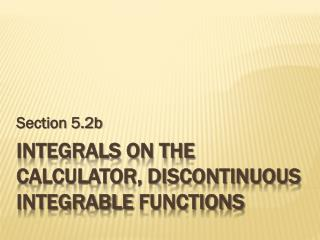DownloadDownload PresentationIntegrals on the Calculator, discontinuous integrable functions

# Integrals on the Calculator, discontinuous integrable functions

Download Presentation## Integrals on the Calculator, discontinuous integrable functions

- - - - - - - - - - - - - - - - - - - - - - - - - - - E N D - - - - - - - - - - - - - - - - - - - - - - - - - - -
##### Presentation Transcript

1. Section 5.2b Integrals on the Calculator, discontinuous integrable functions

2. Do Now: Exploration 1 on page 264 It is a fact that With this information, determine the values of the following integrals. Explain your answers (use a graph, when necessary). 1. 4. 2. 5. 3. 6.

3. Do Now: Exploration 1 on page 264 It is a fact that With this information, determine the values of the following integrals. Explain your answers (use a graph, when necessary). 7. 9. 8. 10. Suppose k is any positive number. Make a conjecture about

4. A Similar Challenge: #29-38 on p.267-268 Use graphs, your knowledge of area, and the fact that to evaluate the given integrals. 29. 32. 30. 33. 31. 34.

5. A Similar Challenge: #29-38 on p.267-268 Use graphs, your knowledge of area, and the fact that to evaluate the given integrals. 35. 37. 36. 38.

6. Integrals on the Calculator Our modern calculators are good at calculating Riemann sums…our text denotes this function as NINT: We write this statement with an understanding that the right- hand side of the equation is an approximation of the left-hand side…

7. Integrals on the Calculator Examples: Evaluate the following integrals numerically.

8. Discontinuous Integrable Functions As we already know, a function is not differentiable where it is discontinuous. However, we can integrate functions that have points of discontinuity. Examples… Let’s look at the graph… Find 1 Discontinuity at x = 0!!! –1 1 2 –1 Areas of rectangles: What does our calculator give us on this one???

9. Discontinuous Integrable Functions As we already know, a function is not differentiable where it is discontinuous. However, we can integrate functions that have points of discontinuity. Examples… Explain why the given function is not continuous on [0, 3]. What kind of discontinuity occurs? Removable discontinuity at x = 2

10. Discontinuous Integrable Functions As we already know, a function is not differentiable where it is discontinuous. However, we can integrate functions that have points of discontinuity. Examples… Use areas to show that The thin strip above x = 2 has zero area, so the area under the curve is the same as A Trapezoid!!!

11. Discontinuous Integrable Functions As we already know, a function is not differentiable where it is discontinuous. However, we can integrate functions that have points of discontinuity. Examples… Use areas to show that Sum the rectangles: